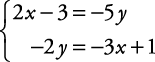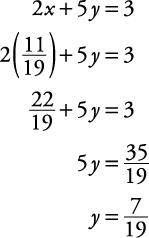## Linear Equations: Solutions Using Elimination with Two Variables

To solve systems using elimination, follow this procedure.

• Arrange both equations in standard form, placing like variables and constants one above the other.

• Choose a variable to eliminate, and with a proper choice of multiplication, arrange so that the coefficients of that variable are opposites of one another.

• Add the equations, leaving one equation with one variable.

• Solve for the remaining variable.

• Substitute the value found in Step 4 into any equation involving both variables and solve for the other variable.

• Check the solution in both original equations.

##### Example 1

Solve this system of equations by using elimination.Arrange both equations in standard form, placing like terms one above the other.Select a variable to eliminate, say y.

The coefficients of y are 5 and –2. These both divide into 10. Arrange so that the coefficient of y is 10 in one equation and –10 in the other. To do this, multiply the top equation by 2 and the bottom equation by 5.Add the new equations, eliminating y.Solve for the remaining variable.Substitute for x and solve for y.Check the solution in the original equation.These are both true statements. The solution is.

If the elimination method produces a sentence that is always true, then the system is dependent, and either original equation is a solution. If the elimination method produces a sentence that is always false, then the system is inconsistent, and there is no solution.